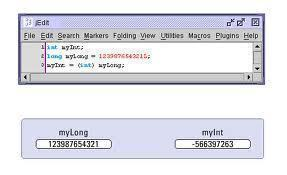•• # Type Conversion – Class to Class

Now that we have understood how to convert basic data types to class types and vice-versa, it is time to learn how to convert objects of one class type to another class type.

The conversion between objects of different classes can be done using either a one-argument constructor or a conversion function. The choice depends upon whether the conversion routine has to be declared in the source class or in the destination class. To illustrate, consider a program that contains two classes: A and B. Also consider the statement:

object_A = object_B;

In the above statement, object_B of type B is converted to type A and assigned to object_A. Therefore, object_B is the source and object_A is the destination.

For the conversion, the user can use either a conversion function or a constructor with one argument, depending on whether it is specified in the source class or the destination class. In other words, if class B handles the conversion, it will hold a conversion function. On the other hand, if class A carries out the conversion, it will do that through a constructor that takes an argument of type class B.Note that only one of the two conversion routines should be specified; the compiler will yield an error if both are specified since it will not be able to figure out which routine to call.

## Sample Program

.cf { font-family: Lucida Console; font-size: 9pt; color: black; background: white; }
.cl { margin: 0px; }
.cb1 { color: green; }
.cb2 { color: blue; }
.cb3 { color: maroon; }

`/*`
` * Program converts from one class type to`
` * another using a conversion function`
` * and a constructor`
` * https://www.tech-faq.com`
` */`
`#include <iostream>`
`using namespace std;`
`class Kilometers`
`{`
`private:`
`    double kilometers;`
`public:`
`    Kilometers(double kilometers): kilometers(kilometers) {}`
`    void display()`
`    {`
`        cout << kilometers << " kilometeres";`
`    }`
`    double getValue()`
`    {`
`        return kilometers;`
`    }`
`};`
`class Miles`
`{`
`private:`
`    double miles;`
`public:`
`    Miles(double miles) : miles(miles) {}`
`    void display()`
`    {`
`        cout << miles << " miles";`
`    }`
`    operator Kilometers()`
`    {`
`        return Kilometers(miles*1.609344);`
`    }`
`    Miles(Kilometers kilometers)`
`    {`
`        miles = kilometers.getValue()/1.609344;`
`    }`
`};`
`int main(void)`
`{`
`    /*`
`    * Converting using the conversion function`
`    */`
`    Miles m1 = 100;`
`    Kilometers k1 = m1;`
`    m1.display();`
`    cout << " = ";`
`    k1.display();`
`    cout << endl;`
`    /*`
`    * Converting using the constructor`
`    */`
`    Kilometers k2 = 100;`
`    Miles m2 = k2;    // same as:  Miles m2 = Miles(k2);`
`    k2.display();`
`    cout << " = ";`
`    m2.display();`
`    cout << endl;`
`}`

### Output

`100 miles = 160.934 kilometeres`
`100 kilometeres = 62.1371 miles`

## In this Series

1. #### Vikas

30 November, 2015 at 11:23 am

what does this line means ” Kilometers(double kilometers): kilometers(kilometers) {}”?